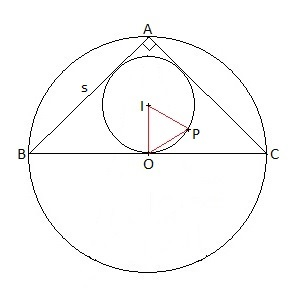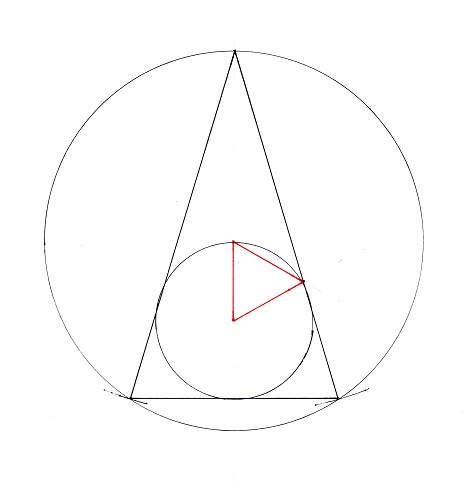# »  Solutions to puzzles in my VDARE.com monthly Diary

##June 2021

—————————

In the Math Corner of my June Diary I included the following brainteaser.

Bailey's Triangle.

This month's brainteaser is from the "Problems" section of the May 2021 College Mathematics Journal. As is usual with these journals, it'll be a few months before they publish a worked solution.

It was proposed by Herb Bailey of the Rose-Hulman Institute of Technology in Terre Haute, Indiana. With a respectful nod to the shade of Frank Morley, I hereby christen it the problem of Bailey's Triangle.

An isosceles triangle has incenter I, circumcenter O, side length [i.e. for each of the two equal sides] s, and base length w. Show that there is a unique value of s/w so that there exists a point P on one of the two equal sides such that triangle IOP is equilateral. Find this value.

This is another one of those problems I've diddled with for half an hour on and off through the month, but never solved to my own full satisfaction. I mean, I solved it in the sense of figuring out the answer, but I did so by the breaking-rocks method, i.e. brute-force algebra. I feel sure there is a much smarter, more elegant geometric proof, but I couldn't find it.

—————————

•  Solution

• The case u = 1/√2.

It helps to think of the problem as one in which everything is happening in the circumcircle.In the diagram shown, for example, the isosceles triangle is the special case of one with a right angle at the apex. Here AB and AC both have length s, BC has length w, and s/w = 1/√2, which is 0.70710678 and change.

Since the number s/w is what interests us, I'll call it u for simplicity and stop referring to w altogether. When w turns up in the algebra, I'll just replace it with s/u. So this diagram shows the case u = 0.70710678

An important thing to notice about u is that it must be greater than ½. If u were to have the value exactly ½, then AB and AC together would add up to precisely BC, and the triangle ABC would have degenerated into a line segment of zero area. So for there to be a triangle at all we must have u > 0.5.

For the right-angled case shown, u is 1/√2, as already stated. The circumradius AO (or BO or CO) is clearly s/2u (remember I'm replacing w with s/u), or 0.70710678s. The inradius IO needs some basic trig; it works out to (1 − 1/√2)s, which is 0.29289321s.

In this particular case the geometry of the situation puts P, the apex of Bailey's Triangle, actually on the incircle. P is, however, nowhere near AC, which is what we are looking for.

Let's try some different values of u.

• The case 0.5 < u < 0.70710678.

Values of u between the case shown there, u = 0.70710678, and the lower bound of u = 0.5, don't look promising, although I confess I haven't done the math. Decreasing u slides the base BC upwards, making the triangle ABC "flatter," towards the degenerate case. (While O heads off to infinity southwards.)

With u < 0.70710678, BC is no longer a diameter of the circumcircle; and I, the incenter of triangle ABC, migrates north relative to the circumcenter O. That makes for an ever-bigger Bailey's Triangle; but AC is swinging northwards at the same time as P is heading east, but also south … Not promising for having P meet AC.

So let's try increasing the value of u.

• The case u = 1.

Increasing u from the value u = 0.70710678, the triangle ABC gets "skinnier." The incenter I moves southwards towards O.

At u = 1 the triangle ABC is actually equilateral. I now coincides with O, and Bailey's Triangle has dwindled to one single point there at the center of triangle ABC.

• The case u = 2.If we continue to increase u, I moves south of O and the Bailey Triangle reappears. The diagram here shows the case u = 2.

At u = 1 the Bailey Triangle had dwindled to a single point. At u = 2 it has reappeared and increased in size to where the apex P has broken through AC.

Plainly there is some value of u between 1 and 2 for which P lies on AC. That is to say, using the labels in my diagram, when

MK = MP.

What we have to do is turn that into an equation in u, then solve the equation.

• The general case when u is in the neighborhood of 2.

Plainly MP is just ½√3 times OI. What about MK?

AMK and AHC are similar triangles, so MK/AM = HC/AH. That gives us MK = AM×HC/AH. Further, AM is AO plus OI/2. So our equation MK = MP now looks like this:

(AO + OI/2)×HC/AH = (½√3)×OI.

Cleaning up a bit:

2AO×HC + OI×HC = √3×OI×AH.

And then a bit more:

(√3×AH − HC) × OI − 2AO × HC = 0.

That's the equation to solve; and at this point, there is nothing for it but to work out the lengths AH, HC, OI, and AO in terms of s and u. This is where the rock-breaking starts.

HC is the easiest one. It's just half of w, and so it's s/(2u).

AH is not much harder. By Pythagoras it's the square root of s² − s²/(4u²), which simplifies — well, a bit — to (s/(2u))√(4u² − 1).

Now things get knottier. AO we can work out by dropping a perpendicular from circumcenter O to chord AB. Calling the foot of that perpendicular X, AX is half of AB. By similar triangles AXO, AHB, we get AO/AX = AB/AH. That boils down to AO = su/√(4u² − 1).

OI is AH − AO − IH. We've just figured out AH and AO. To get IH we drop a different perpendicular, this time from I to AB. I'll call the foot of that perpendicular Y.

Looking at similar triangles AIY, ABH, we have IY/AY = BH/AH. Since both IY and IH are radii of the incircle, they are equal. BY is equal to BH, which is s/(2u), so that AY = s − s/(2u); AH we have already figured out. Putting it all together, IH = (s/(2u))(2u − 1) / √(4u² − 1).

Now we have AH, AO, and IH, so we can calculate OI. It works out to s(u − 1) / √(4u² − 1).

Now we have AH, HC, OI, and AO all in terms of s and u, so we can go ahead and rewrite the equation we need to solve, the one that started out as MK = MP.

To state the equation in its last form once again, it's

(√3×AH − HC) × OI − 2AO × HC = 0.

Substituting the values we've worked out, this becomes

(√3×(s/(2u))√(4u² − 1) − (s/(2u))) × s(u − 1) / √(4u² − 1) − (2su/√(4u² − 1)) × (s/(2u)) = 0

It looks ugly; but if I just multiply throughout by 2u(√(4u² − 1))/s²  it simplifies wonderfully to:

(√3 × √(4u² − 1) − 1) × (u− 1) − 2u = 0

With some rearranging and a squaring, that becomes a straightforward quartic equation:

3u4 − 6u3 + 3u − 1 = 0

No seasoned math warrior will be deterred by a mere quartic. Divide through by 3 to simplify:

u4 − 2u3 + u − 1/3 = 0

Substitute t = (u − 1/2) to get rid of the cubic term;

t4 − (3/2)t2 − 1/48 = 0

That's just a quadratic in t2. Solving, taking the more obvious root, and and substituting back for u:

u = (1 + √(3 + 2√(7/3))) / 2.

That works out to u = 1.7303506068702… and a standard quartic equation app confirms.

Now my brain's numb from all that rock-breaking, so I need to go lie down.

Added later:  In an idle moment I dug out my ruler & compass and did the best I could with that value of u. My draftsmanship is not all it could be, but sure enough I got a Bailey's triangle just kissing AC.It looks as though OI is exactly equal to IH here. That's not in fact the case. When I crunched the numbers for this configuration, with s = 15.31746463cm. and w = 8.85223178cm., I got OI = 3.37665954cm. but IH = 3.28738564cm., so that OI is longer by 2.7 percent than IH.

When OI actually is equal to IH, u = 1.707106781, which is to say u = 1 + 1/√2.

So there's fun stuff happening around u = 1.7. That raises my suspicions, though. The square root of 3 is 1.7320508075… Is u = √3 the correct answer, with these other 1.7-somethings just by-products of some tiny slip in my algebra back there? I wouldn't rule it out